Two Lines of Symmetry

Two Lines of Symmetry - Line of Symmetry Definition

The balanced and proportionate similarity between two halves of an object is termed as Symmetry. In other words, one half is the mirror image of another half. It is must to understand the concept of line of symmetry. For example, when you fold the heart shape paper, the paper is folded from the center to get the perfect symmetry of the heart. When you open the folded heart, you can find the line on the center of the heart from where it is cut into two halves. This file which cuts the heart into two equal parts is known as the Line of Symmetry.

A figure which does not have any similarity between two halves is known as a Asymmetrical Figure. In an Asymmetrical figure, none of the sides of the shape is equal.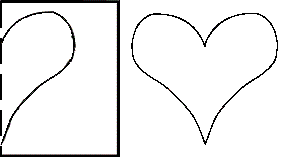You cannot fold the heart further to get the perfect symmetry. Hence, we can say that the heart-shape has one and only one line of Symmetry. There are some figures wherein the lines of symmetry can be more than one. It is very important to understand the following points before studying the line of Symmetry in details.

• 1. If a figure or shape does not have a line of symmetry, it indicates that the shape or figure is asymmetrical.

• 2. Few shapes or figures have one line of symmetry, two lines of symmetry or multiple lines of symmetry.

• A circle can have infinite number of lines of symmetry as it can be folded at any diameter to get the symmetrical halves. A square is said to have four lines of symmetry as its side are the same so the diagonal lines and vertical as well as horizontal lines divide a square. The equilateral triangle has three lines of symmetry whereas a scalene triangle does not have any line of symmetry as its all sides are different. An isosceles triangle has one line of symmetry as both of its sides are equal. A Pentagon has 5 lines of Symmetry and a Hexagon has six lines of symmetry.

Two Lines of Symmetry: Some figure can be divided into two equal parts with two lines. These shapes are said to have Two Lines of Symmetry. The rectangle is an example of Two Line of Symmetry. A Rectangle can be divided vertically, horizontal or diagonally to get the two symmetrical parts. A rhombus also has two lines of symmetry. Two Lines of Symmetry can be a combination of Vertical, diagonal or Horizontal Lines. Letters such as H and X also have two lines of Symmetry. An hourglass is also an example of Two Lines of Symmetry.
A rectangle does not have a diagonal line of symmetry as opposed to square as its sides are not the same. The rectangle can only have a vertical and horizontal line of symmetry.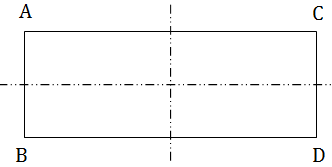In this figure, you can see that the rectangle is divided into equal parts vertically and horizontally. But if you draw a diagonal line from A to D, you can see that the sides will not match. You can try this with a piece of paper as well.

To understand the concept of Symmetry, let us take up an activity:

Take a piece of A4 size of paper, and hold it vertically to get two equal halves. Then fold it again vertically. Now you can also draw a picture and cut the same. When you open the cutout picture, you will notice that the design you made also has two lines of Symmetry.

One can also go to a supermarket and observe the lines of symmetry of different objects such as boxes, packages, containers etc. You can also observe the fruits and vegetables around and try to find the line of symmetry of these various objects.

Problems on Line of Symmetry:

• 1. Give four examples of symmetrical objects from your home or at school?

• Answer: Inkpot, Notebook, Glass, Backboard.

• 2. Identify the mirror line in the figure given below?

• Answer: l2 as it divides the shape into two equal parts. The line of symmetry is the line which divides a shape or a figure in two equal parts.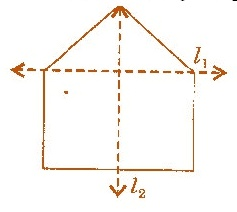3. Complete the diagram and make it look symmetrical. In the shape given below L is the line of symmetry.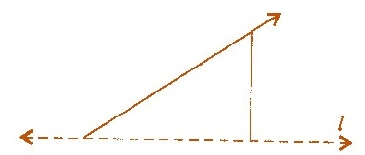Answer: The line of symmetry divides the shape into two equal parts. Hence, the mirror image of this shape will complete the diagram.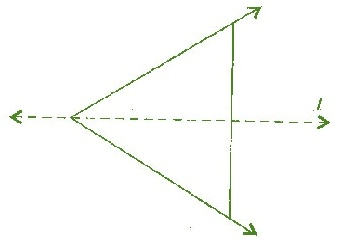• 4. In the figure given below L is the line of symmetry. Draw the triangle to complete the diagram so that it looks symmetric.

•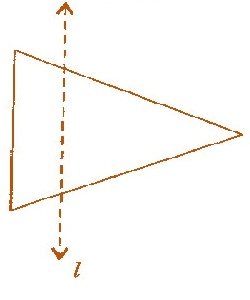Answer: Adding a mirror image will complete the figure given above and make it look symmetrical. The line of symmetry divides the shape into two equal halves; hence the shape that one gets is given below: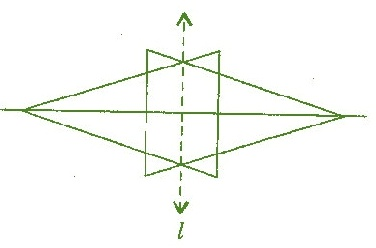• 5. List the number of symmetry lines in each of the shapes given below:

•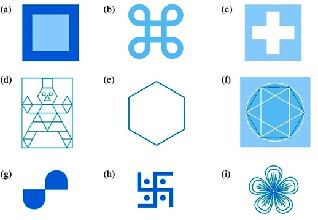• (a) In figure A, there are four lines of symmetry.

• (b) In Figure B, there are four lines of symmetry.

• (c) In Figure C, there are four lines of symmetry.

• (d) In figure D, there are no lines of symmetry.

• (e) In figure E, there are 6 lines of symmetry.

• (f) In figure F, there are 6 lines of symmetry.

• (g) In figure G, there are no lines of Symmetry.

• (h) In figure H, there are no lines of symmetry.

• (i) In figure I, there are 3 lines of Symmetry.

• 6. Can one draw a triangle having one line of Symmetry?

• Answer: Yes, an Isosceles triangle has one line of symmetry as both its sides are equal.
• 7. Can you draw a triangle with three lines of Symmetry?

• Answer: Yes, an Equilateral triangle. The equilateral triangle has three lines of symmetry as all of its three sides are equal.
• 8. Make a list of Lines of symmetry in Alphabets from A to Z. Divide them as per their lines of symmetry that is vertical, horizontal, no line of symmetry.